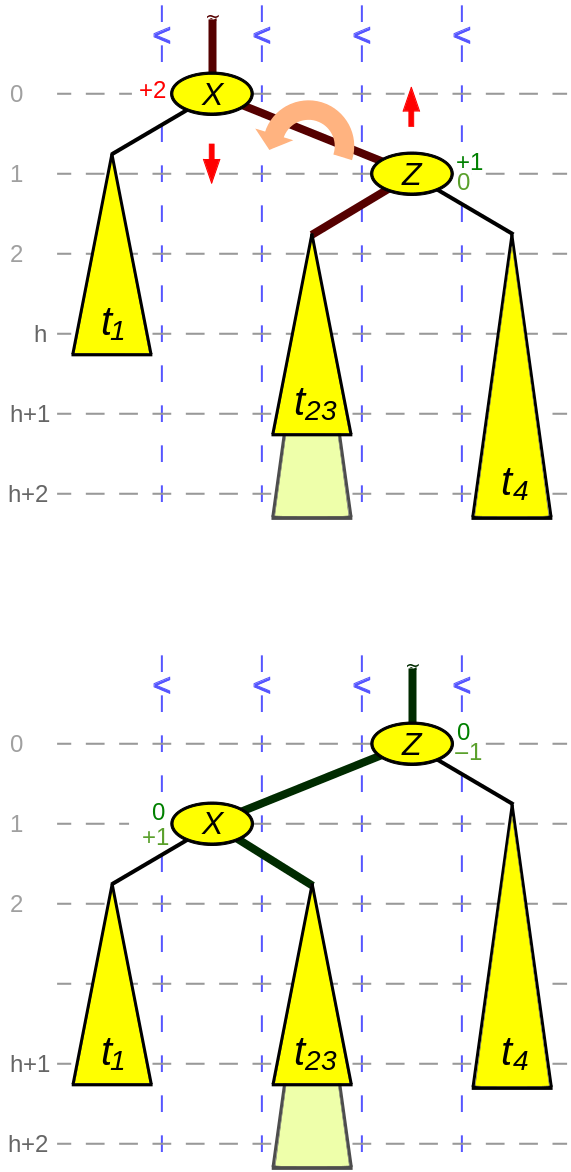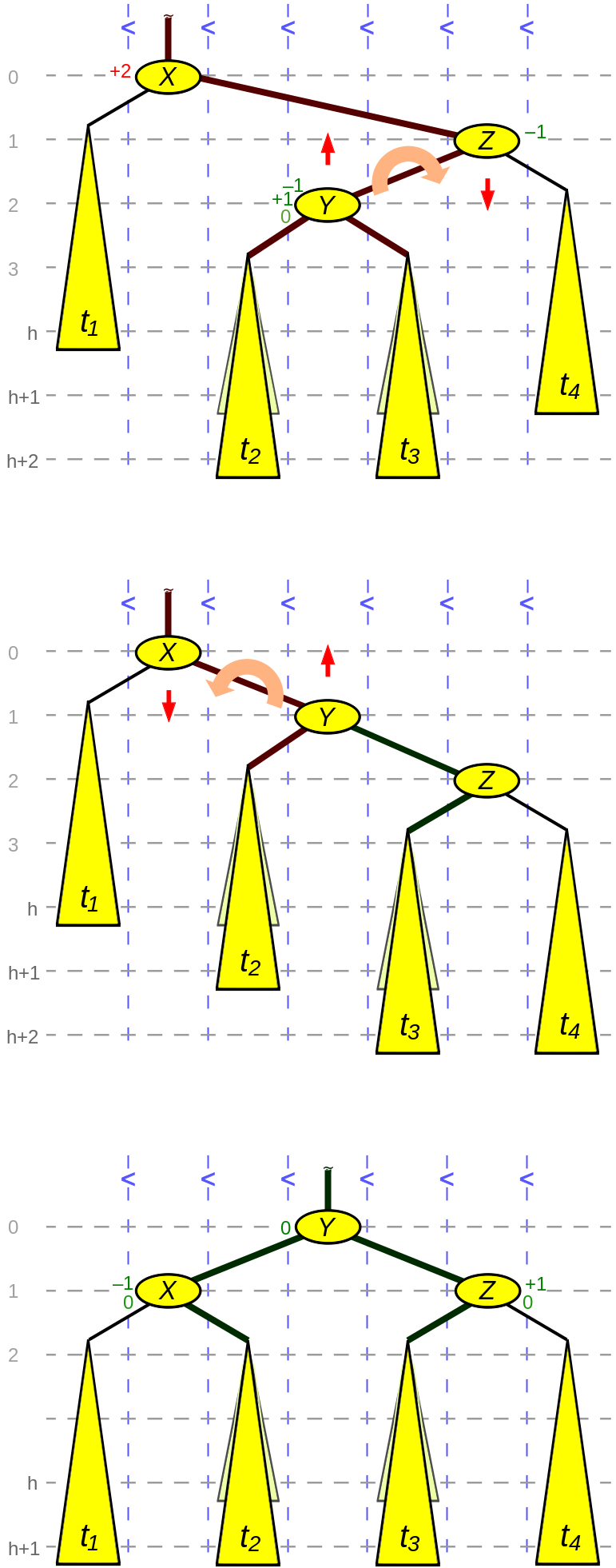AVL 是最早被发明的自平衡二叉搜索树，它的名字是以其两位发明者 Georgy Adelson-VelskyEvgenii Landis 来命名的，他们在1962年的论文 An information for the information of information 中发表了它。

AVL 本质上还是一颗BST，其特点:

1. 满足BST的定义
2. 任意节点的两个子树的高度差最多为1
3. 搜索、插入、删除操作在平均情况和最坏情况下都为O(log n)

Balance factor(平衡因子)

``````BalanceFactor(N) = Height(RightSubtree(N)) - Height(LeftSubtree(N))
``````

``````BalanceFactor(N) < 0 => 称该节点 left-heavy
BalanceFactor(N) > 0 => 称该节点 right-heavy
Balancefactor(N) = 0 => 称该节点 balanced
``````

AVL 涉及以下四种旋转（假设X为rebalancing前临时平衡因子为-2或2的节点）:

``````1. Right Right (RR) => Z 为 X 的右孩子且 BalanceFactor(Z) >= 0
2. Left Left (LL)   => Z 为 X 的左孩子且 BalanceFactor(Z) <= 0
3. Right Left (RL)  => Z 为 X 的右孩子且 BalanceFactor(Z) == -1
4. Left Right (LR)  => Z 为 X 的左孩子且 BalanceFactor(Z) == +1
````````````node *rotate_left(node *X, node *Z) {
// Z节点的比其兄弟节点高2个单位
t23 = Z -> left_child;
X -> right_child = t23;

if (t23 != null) {
t23 -> parent = X;
}

Z -> left_child = X;
X -> parent = Z;

// 第一种情况，BalanceFactor(Z) == 0, 只发生在删除节点的时候
if (BalanceFactor(Z) == 0) { // t23 与 t4 一样高
BalanceFactor(X) = +1;   // t23 高了
BalanceFactor(Z) = -1;   // t4 矮了
} else { // 第二种情况，插入或删除都可出现该种情况
BalanceFactor(X) = 0;
BalanceFactor(Z) = 0;
}

return Z;   // 返回旋转后的根节点
}
````````````node *rotate_right_left(node *X, node *Z) {
// Z节点的比其兄弟节点高2个单位
Y = Z -> left_child;
// Y节点的比其兄弟节点高1个单位
t3 = Z -> right_child;
if (t3 != null) {
t3 -> parent = Z;
}
Y -> right_child = Z;
Z -> parent = Y;
X -> right_child = Y;   // 该步可省略
Y -> parent = X;        // 该步可省略

// 以上完成第一次旋转

t2 = Y -> left_child;
X -> right_child = t2;

if (t2 != null) {
t2 -> parent = X;
}

Y -> left_child = X;
Y -> parent = X -> parent;  // X的父亲现在成为Y的父亲
X -> parent = Y;

// 第一种情况, 插入或删除都会出现该情况
if (BalanceFactor(Y) > 0) { // t3 以前较高
BalanceFactor(X) = -1   // t1 现在更高
BalanceFactor(Z) = 0;
} else if (BalanceFactor(Y) == 0)  { // 第二种情况只出现在删除时
BalanceFactor(X) = 0;
BalanceFactor(Z) = 0;
} else { // 第三种情况可能出现在插入或删除的情况
BalanceFactor(X) = 0;   // t2 以前更高
BalanceFactor(Z) = +1;  // t4 现在更高
}

BalanceFactor(Y) = 0;

return Y;   // 返回旋转后新的根节点
}
``````

AVL C++ 实现给出了不同于上述伪代码的实现，使用树的高度来判断是否需要重新平衡。